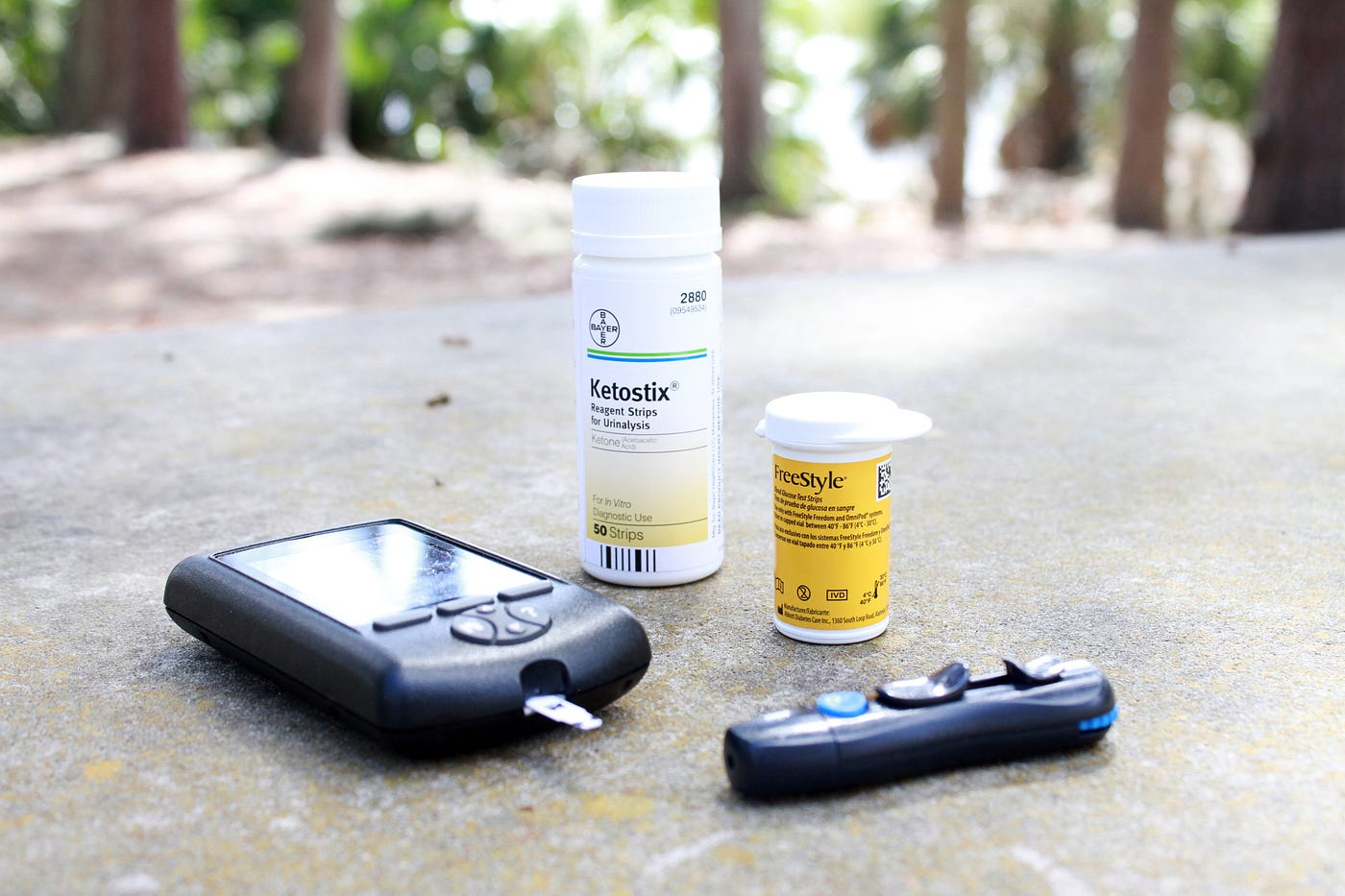# Predicting Readmission within 30 days for diabetic patients with TensorFlowPhoto by Mykenzie Johnson on Unsplash

# Basics of deep learning

To understand how deep learning works, lets go through how we as humans learn. For example, let’s say that we wanted to cook something. We’d get the ingredients, cook and taste it to see how good our recipe tastes. After doing this over and over and every try making subtle changes that make the recipe taste better you finally perfect the recipe. Deep learning works in the same way. You give the model data, the model makes connections with the data by adding biases and weights then the model gives a predicted output. We measure how incorrect the model was and let the model try again, using what it learns from the previous attempts and tweaking itself until it achieves the lowest error rate that it can find.

## Hidden layers and activation functions

More hidden layers in a network allows the model to create more complex relationships between the data. This takes away the need for feature extraction in a data set. All the layers in a network are interconnected as you can see in the diagram above. At each node in a hidden layer, you apply what’s called an activation function. These functions are just mathematical functions that get applied to values in the nodes of a hidden layer and are the reason why hidden layers can make complex non-linear connections. For a deeper understanding check this out.

# Making our model

Now that you have a basic understanding of deep learning, let’s get started on building out the model.

## Data preparation

We’re going to be using NumPy and pandas so lets go ahead and import them.

`import pandas as pdimport numpy as np`
`df = pd.read_csv("diabetic_data.csv")df.info()df.head()`
`# drop columns not neededdf[df == "?"] = np.nandf = df.drop(["encounter_id","weight", "medical_specialty","patient_nbr"],axis=1)`
`def binary_readmitted(elem):    if(elem == "<30"):        return 1    return 0df["readmitted"] = df["readmitted"].apply(binary_readmitted)`
`string_columns = [    "race",    "gender",    "age",    "max_glu_serum",    "A1Cresult",    "metformin",    "repaglinide",    "payer_code",    "diag_1",    "diag_2",    "diag_3",    "nateglinide",    "chlorpropamide",    "glimepiride",    "acetohexamide",    "glipizide",    "glyburide",    "tolbutamide",    "pioglitazone",    "rosiglitazone",    "acarbose",    "miglitol",    "troglitazone",    "tolazamide",    "examide",    "citoglipton",    "insulin",    "glyburide-metformin",    "glipizide-metformin",    "glimepiride-pioglitazone",    "metformin-rosiglitazone",    "metformin-pioglitazone",    "change",    "diabetesMed"]df_dummies = pd.get_dummies(df[string_columns],drop_first=True)df = df.drop(string_columns,axis=1)`
`df_dummies`
`df = df.join(df_dummies)`
`readmit_patients = df[df["readmitted"] == 1][:10500]not_readmit_patients = df[df["readmitted"] == 0][:10500]balanced_df = readmit_patients.append(not_readmit_patients)`
`y = balanced_df["readmitted"].valuesX = balanced_df.drop("readmitted", axis=1).valuesX_train, X_test, y_train, y_test = train_test_split(X,y, random_state=42, test_size=0.3)input_shape = (len(list(df.columns)) - 1,)`

## Building and training our model

For our model, we’re going to use Tensorflow Keras. Our model is going to be sequential, meaning that one layer comes after another. We’re going to have An input layer with 120 nodes and a ReLU activation; 1 hidden layer with 50 nodes and ReLU activation and finally our output layer with 1 node for one output and a Sigmoid activation because we’re doing binary classification. If you’re interested in going deeper into configuring the model architecture check this out.

`model = Sequential()model.add(Dense(120, activation='relu', input_shape=input_shape))model.add(Dense(50, activation='relu'))model.add(Dense(1, activation='sigmoid'))early_stopping_monitor = EarlyStopping(patience=2)`
`model.compile(loss='binary_crossentropy', optimizer='adam', metrics=['accuracy'])`
`history = model.fit(X_train,y_train,epochs=13, validation_split=0.3, batch_size=10,          callbacks=[early_stopping_monitor]         )`

## Evaluating our model

Doing this we get a validation accuracy of 0.78 and a validation loss of 0.41. Let’s look at the true positives. True positives are when the model predicts that the patient will be readmitted within 30 days and the prediction is true. We measure our true positives with AUC, the area under the curve of true positives over false positives. The closer to 1, the better and the closer to 0.5 the more the model is predicting randomly.

`from sklearn.metrics import roc_curve, aucimport matplotlib.pyplot as plty_pred_test = model.predict(X_test)[:,0]y_pred_train = model.predict(X_train)[:,0]fpr_test, tpr_test, thresholds_test = roc_curve(y_test, y_pred_test)fpr_train, tpr_train, thresholds_train = roc_curve(y_train, y_pred_train)auc_test = auc(fpr_test, tpr_test)auc_train = auc(fpr_train, tpr_train)plt.figure(1)plt.plot([0, 1], [0, 1], 'k--')plt.plot(fpr_train, tpr_train, label='Train (area = {:.3f})'.format(auc_train))plt.plot(fpr_test, tpr_test, label='Test (area = {:.3f})'.format(auc_test))plt.xlabel('False positive rate')plt.ylabel('True positive rate')plt.title('ROC curve')plt.legend(loc='best')plt.show()`
`plt.plot(history.history['loss'])plt.plot(history.history['val_loss'])plt.title('model loss')plt.ylabel('loss')plt.xlabel('epoch')plt.legend(['train', 'val'], loc='upper left')plt.show()`

--

--